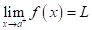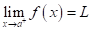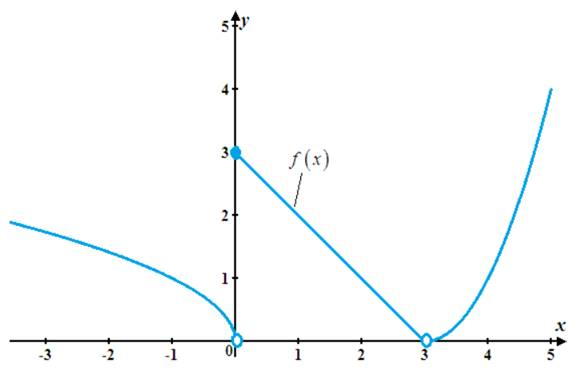# The value of limits.### Single Variable Calculus: Concepts...

4th Edition
James Stewart
Publisher: Cengage Learning
ISBN: 9781337687805### Single Variable Calculus: Concepts...

4th Edition
James Stewart
Publisher: Cengage Learning
ISBN: 9781337687805

#### Solutions

Chapter 2, Problem 23RE

(a)

To determine

## To find:The value of limits.

Expert Solution

The value for each limit is calculated.

### Explanation of Solution

Given information:

The given function is f(x)={xifx<03xif0x<3(x3)2ifx>3

Calculation:

(i) Calculate the value of limx0+f(x) .

For x>0 , the function is f(x)=3x .

limx0+f(x)=limx0+(3x)=30=3

(ii) Calculate the value of limx0f(x) .

For x<0 , the function is f(x)=(x) .

limx0f(x)=limx0(x)=(0)=0

(iii) Calculate the value of limx0f(x) .

The left limit limx0f(x)=0 and right limit limx0+f(x)=3 are not same.

Recollect the result

limxaf(x)=L if and only ifand limxaf(x)=L .

Using the result, the limit of the function f(x) does not exist at x=0 .

So, the value of limx0f(x) does not exist.

(iv) Calculate the value of limx3f(x) .

For x<3 , the function is f(x)=3x .

limx3f(x)=limx3(3x)=3(3)=0

So, the limit for limx3f(x) is equal to zero.

(v) Calculate the value of limx3+f(x) .

For x>3 , the function is f(x)=(x3)2 .

limx3+f(x)=limx3+(3x)=(3(3))2=0

So, the limit for limx3+f(x) is equal to zero.

(vi) Calculate the value of limx3f(x) .

The left limit limx3f(x)=0 and right limit limx3+f(x)=0 are same.

Recollect the result

limxaf(x)=L if and only ifand limxaf(x)=L .

Using the result, the limit of the function limx3f(x)=0

So, the value of limx3f(x) is equal to zero.

Therefore, the value for each limit is calculated.

(b)

To determine

### To find:The discontinuous function f .

Expert Solution

The function has discontinuous at x=0 and x=3 .

### Explanation of Solution

Given information:

The given function is f(x)={xifx<03xif0x<3(x3)2ifx>3

Calculation:

The function is said to be discontinuous at x , if the function does not continuous at x .

Using the part (iii) , limx0f(x)=doesnotexist and f(0)=3 .

limx0f(x)f(0)

Therefore, the function f(x) is not continuous at x=0 .

Using the part (vi) , limx3f(x)=0 and f(3) is not defined.

limx3f(x)f(3)

Hence, the function is not continuous at x=0 .

Therefore, the function has discontinuous at x=0 and x=3 .

(c)

To determine

Expert Solution

### Explanation of Solution

Given information:

The given function is f(x)={xifx<03xif0x<3(x3)2ifx>3 .

Graph:

The graph for the given function is shown in figure (1).Figure (1)

Interpretation: Graph for the function f is shown in figure (1).

### Have a homework question?

Subscribe to bartleby learn! Ask subject matter experts 30 homework questions each month. Plus, you’ll have access to millions of step-by-step textbook answers!